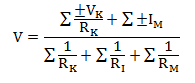# MCQs on Electric Circuits

##### Page 12 of 63. Go to page 1 2 3 4 5 6 7 8 9 10 11 12 13 14 15 16 17 18 19 20 21 22 23 24 25 26 27 28 29 30 31 32 33 34 35 36 37 38 39 40 41 42 43 44 45 46 47 48 49 50 51 52 53 54 55 56 57 58 59 60 61 62 63
01․ For a band pass filter, cut off frequency of the low pass filter should be ___ of the high pass filter?
greater than
less than
equals to
all of the above

Band pass filter is obtained with the combination of LPF and HPF, and cut off frequency of LPF should be less than the cut off frequency of HPF.

02․ Discrete values represent which type of signal
Analog
Discretal
Decimal
Digital

A digital signal is represented by discrete values sampled at equal interval of time. It is sampled at particular instant and no in between state is there. It is used in Digital Signal Processing.

03․ Which of the following is not a vector quantity?
Linear momentum.
Angular momentum.
Electric field.
Electric potential.

We know that the electrical potential is E = - ΔVE. It is nothing but the amount of work needed to get a positive charge to that spot. It is a scalar quantity because work is not a vector quantity. However, scalars are allowed to be negative. The minus sign on the potential does not indicate the vector direction. A negative potential can be attracted by a positive potential and repulsed by another negative potential.

04․ The number of independent equations to solve a network is equal to
number of chords.
number of branches.
sum of number of branches and chords.
sum of number of branches, nodes and chords.

The chords are nothing but the group of three or more nodes connected together. Each node has loop equations. That equation is independent to each other. Hence, the number of independent equations to solve a network is equal to its number of chords or otherwise it can be written numerically as b-n+1.

05․ The common voltage across parallel branches with different voltage sources can be determined by the relation V = (V1 / R1 + V2 / R2 + V3 / R3) / (1 / R1 + 1 / R2 +1 / R3 .....) The statement is associated with which theorem?
Superposition theorem.
Thevenin’s theorem.
Norton’s theorem.
Millman’s theorem.

The Millman’s theorem states that the voltage at the ends of the circuit is given byHere, VK is the voltage generators and IM the current generators. RI is the resistances on the branches with no generator. RK is the resistances on the branches with voltage generators. RM is the resistances on the branches with current generators. Hence, the given voltage relation is clearly matched with the above formula. Thus, the statement is associated with the Millman’s theorem. Milliman's theorem can be applied when no of voltage and current sources are acting in parallel.

06․ If conductance increases as temperature increases it is called as ..........
negative co-efficient.
positive co-efficient.
both 1 and 2.
none of the above.

A negative temperature coefficient (NTC) occurs when the thermal conductivity of a material rises and resistivity decreases with increasing temperature, typically in a defined temperature range.

07․ What is mean by flow of electrons?
Insulator.
Electric current.
Semiconductor.
Voltage.

Conventional flow of electric current is the opposite of flow of electrons.

08․ Which material has extremely large resistance?
Semiconductor
Conductor
Insulator
None of the above

An electrical insulator is a material whose internal electrons do not flow freely. Insulators have higher resistivity ( ≈ 10-15 ) than semiconductors or conductors.

09․ What is meant by the rate of flow of an electric charge?
Electric current.
Electric potential.
Electric resistance.
None of the above.

The rate of flow of electric charge per unit time is defined as electric current.

10․ What is the unit of potential difference ?
Ohm
Volt
Watt
Ampere

Potential difference between the two points is also known as voltage. For open circuit it is called potential difference and for closed circuit it is called voltage. The unit of potential difference and voltage the S.I. unit is called Volt.

<<<1011121314>>>